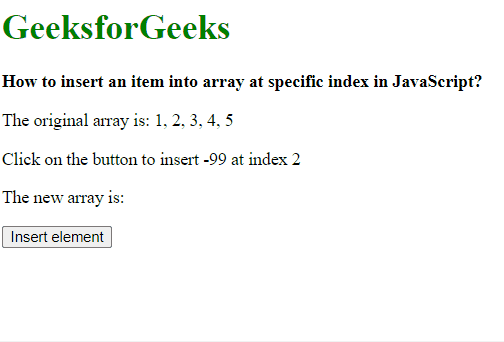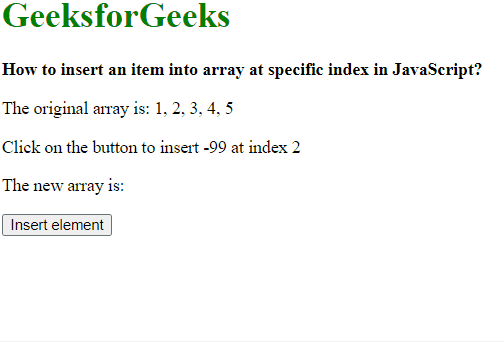GFG App
Open AppBrowser
Continue

# How to insert an item into array at specific index in JavaScript ?

There is no inbuilt method in JavaScript that directly allows for the insertion of an element at any arbitrary index of an array. This can be solved using 2 approaches:

Using array.splice(): The array.splice() method is usually used to add or remove items from an array. This method takes in 3 parameters, the index where the element id is to be inserted or removed, the number of items to be deleted, and the new items which are to be inserted. The only insertion can be done by specifying the number of elements to be deleted to 0. This allows only inserting the specified item at a particular index with no deletion.

Syntax:

`array.splice(index, no_of_items_to_remove, item1 ... itemX)`

Example: In this example, we will insert an element at the 2nd index of the array using the splice() method of Javascript.

## html

 `<``body``>` `    ``<``h1` `style``=``"color: green"``>` `        ``GeeksforGeeks` `    ``` `    ``<``b``>How to insert an item into array` `        ``at specific index in JavaScript?` `    ``<``p``>The original array is: 1, 2, 3, 4, 5` `    ``<``p``>Click on the button to insert -99 at index 2` `    ``<``p``>` `        ``The new array is: <``span` `class``=``"outputArray"``>` `    ```   `    ``<``button` `onclick``=``"insertElement()"``>` `        ``Insert element` `    ``` `    ``<``script` `type``=``"text/javascript"``>` `        ``function insertElement() {` `            ``let arr = [1, 2, 3, 4, 5];` `            ``let index = 2;` `            ``let element = -99;` `        `  `            ``arr.splice(index, 0, element);` `            ``document.querySelector('.outputArray').textContent =` `            ``arr;` `        ``}` `    ``` ``

Output:Using JavaScript for-loop: The for-loop can be used to move all the elements from the index (where the new element is to be inserted) to the end of the array, one place after their current place. The required element can then be placed at the index.

Code:

## javascript

 `// shift all elements one place to the back until index` `for` `(i = arr.length; i > index; i--) {` `arr[i] = arr[i - 1];` `}`   `// insert the element at the index` `arr[index] = element;`

Example: In this example, we will insert an element at the 2nd index of the array using the for loop of Javascript.

## html

 `<``body``>` `    ``<``h1` `style``=``"color: green"``>` `        ``GeeksforGeeks` `    ``` `    ``<``b``>How to insert an item into` `       ``array at specific index in JavaScript?` `    ``` `    ``<``p``>The original array is: 1, 2, 3, 4, 5` `    ``` `    ``<``p``>` `        ``Click on the button to insert -99 at index 2` `    ``` `    ``<``p``>` `        ``The new array is: <``span` `class``=``"outputArray"``>` `    ```   `    ``<``button` `onclick``=``"insertElement()"``>` `        ``Insert element` `    ``` `    ``<``script` `type``=``"text/javascript"``>` `        ``function insertElement() {` `            ``let arr = [1, 2, 3, 4, 5];` `            ``let index = 2;` `            ``let element = -99;` `        `  `            ``// shift all elements one` `            ``// place to the back until index` `            ``for (i = arr.length; i > index; i--) {` `                ``arr[i] = arr[i - 1];` `            ``}` `        `  `            ``// insert the element at the index` `            ``arr[index] = element;` `        `  `            ``document.querySelector(` `            ``'.outputArray').textContent = arr;` `        ``}` `    ``` ``

Output:My Personal Notes arrow_drop_up